# 深度解读ArrayMap优势与缺陷

Posted by Gityuan on January 13, 2019

## 一、引言

ArrayMap是Android专门针对内存优化而设计的，用于取代Java API中的HashMap数据结构。为了更进一步优化key是int类型的Map，Android再次提供效率更高的数据结构SparseArray，可避免自动装箱过程。对于key为其他类型则可使用ArrayMap。HashMap的查找和插入时间复杂度为O(1)的代价是牺牲大量的内存来实现的，而SparseArray和ArrayMap性能略逊于HashMap，但更节省内存。

## 二、源读ArrayMap

### 2.1 基本成员变量

``````public final class ArrayMap<K, V> implements Map<K, V> {

private static final boolean CONCURRENT_MODIFICATION_EXCEPTIONS = true;

private static final int BASE_SIZE = 4;  // 容量增量的最小值
private static final int CACHE_SIZE = 10; // 缓存数组的上限

static Object[] mBaseCache; //用于缓存大小为4的ArrayMap
static int mBaseCacheSize;
static Object[] mTwiceBaseCache; //用于缓存大小为8的ArrayMap
static int mTwiceBaseCacheSize;

final boolean mIdentityHashCode;
int[] mHashes;         //由key的hashcode所组成的数组
Object[] mArray;       //由key-value对所组成的数组，是mHashes大小的2倍
int mSize;             //成员变量的个数
}
``````

1）ArrayMap对象的数据储存格式如图所示：

• mHashes是一个记录所有key的hashcode值组成的数组，是从小到大的排序方式；
• mArray是一个记录着key-value键值对所组成的数组，是mHashes大小的2倍；2）ArrayMap类有两个非常重要的静态成员变量mBaseCache和mTwiceBaseCacheSize，用于ArrayMap所在进程的全局缓存功能：

• mBaseCache：用于缓存大小为4的ArrayMap，mBaseCacheSize记录着当前已缓存的数量，超过10个则不再缓存；
• mTwiceBaseCacheSize：用于缓存大小为8的ArrayMap，mTwiceBaseCacheSize记录着当前已缓存的数量，超过10个则不再缓存。

### 2.2 缓存机制

ArrayMap是专为Android优化而设计的Map对象，使用场景比较高频，很多场景可能起初都是数据很少，为了减少频繁地创建和回收，特意设计了两个缓存池，分别缓存大小为4和8的ArrayMap对象。要理解缓存机制，那就需要看看内存分配(allocArrays)和内存释放(freeArrays)。

#### 2.2.1 freeArrays

``````private static void freeArrays(final int[] hashes, final Object[] array, final int size) {
if (hashes.length == (BASE_SIZE*2)) {  //当释放的是大小为8的对象
synchronized (ArrayMap.class) {
// 当大小为8的缓存池的数量小于10个，则将其放入缓存池
if (mTwiceBaseCacheSize < CACHE_SIZE) {
array = mTwiceBaseCache;  //array指向原来的缓存池
array = hashes;
for (int i=(size<<1)-1; i>=2; i--) {
array[i] = null;  //清空其他数据
}
mTwiceBaseCache = array; //mTwiceBaseCache指向新加入缓存池的array
mTwiceBaseCacheSize++;
}
}
} else if (hashes.length == BASE_SIZE) {  //当释放的是大小为4的对象，原理同上
synchronized (ArrayMap.class) {
if (mBaseCacheSize < CACHE_SIZE) {
array = mBaseCache;
array = hashes;
for (int i=(size<<1)-1; i>=2; i--) {
array[i] = null;
}
mBaseCache = array;
mBaseCacheSize++;
}
}
}
}
``````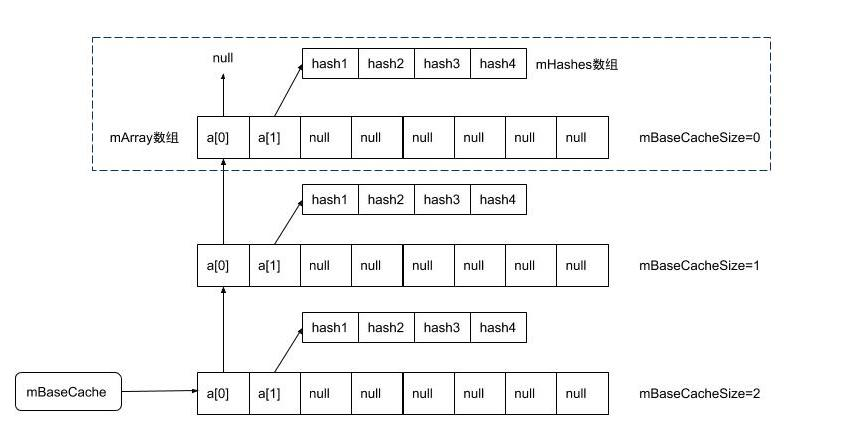freeArrays()触发时机:

• 当执行removeAt()移除最后一个元素的情况
• 当执行clear()清理的情况
• 当执行ensureCapacity()在当前容量小于预期容量的情况下, 先执行allocArrays,再执行freeArrays
• 当执行put()在容量满的情况下, 先执行allocArrays, 再执行freeArrays

#### 2.2.2 allocArrays

``````private void allocArrays(final int size) {
if (size == (BASE_SIZE*2)) {  //当分配大小为8的对象，先查看缓存池
synchronized (ArrayMap.class) {
if (mTwiceBaseCache != null) { // 当缓存池不为空时
final Object[] array = mTwiceBaseCache;
mArray = array;         //从缓存池中取出mArray
mTwiceBaseCache = (Object[])array; //将缓存池指向上一条缓存地址
mHashes = (int[])array;  //从缓存中mHashes
array = array = null;
mTwiceBaseCacheSize--;  //缓存池大小减1
return;
}
}
} else if (size == BASE_SIZE) { //当分配大小为4的对象，原理同上
synchronized (ArrayMap.class) {
if (mBaseCache != null) {
final Object[] array = mBaseCache;
mArray = array;
mBaseCache = (Object[])array;
mHashes = (int[])array;
array = array = null;
mBaseCacheSize--;
return;
}
}
}

// 分配大小除了4和8之外的情况，则直接创建新的数组
mHashes = new int[size];
mArray = new Object[size<<1];
}
``````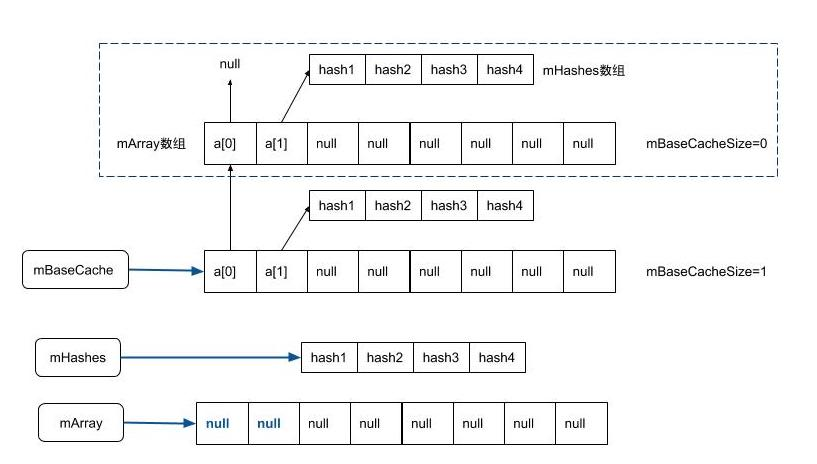allocArrays触发时机:

• 当执行ArrayMap的构造函数的情况
• 当执行removeAt()在满足容量收紧机制的情况
• 当执行ensureCapacity()在当前容量小于预期容量的情况下, 先执行allocArrays,再执行freeArrays
• 当执行put()在容量满的情况下, 先执行allocArrays, 再执行freeArrays

### 2.3 扩容机制

#### 2.3.1 容量扩张

``````public V put(K key, V value) {
...
final int osize = mSize;
if (osize >= mHashes.length) { //当mSize大于或等于mHashes数组长度时需要扩容
final int n = osize >= (BASE_SIZE*2) ? (osize+(osize>>1))
: (osize >= BASE_SIZE ? (BASE_SIZE*2) : BASE_SIZE);
allocArrays(n);  //分配更大的内存【小节2.2.2】
}
...
}
``````

• 当map个数满足条件 osize<4时，则扩容后的大小为4；
• 当map个数满足条件 4<= osize < 8时，则扩容后的大小为8；
• 当map个数满足条件 osize>=8时，则扩容后的大小为原来的1.5倍；

#### 2.3.2 容量收紧

``````public V removeAt(int index) {
final int osize = mSize;
final int nsize;
if (osize > 1) {  //当mSize大于1的情况，需要根据情况来决定是否要收紧
nsize = osize - 1;
if (mHashes.length > (BASE_SIZE*2) && mSize < mHashes.length/3) {
final int n = osize > (BASE_SIZE*2) ? (osize + (osize>>1)) : (BASE_SIZE*2);
allocArrays(n); // 分配更小的内存【小节2.2.2】
}
}
}
``````

• 如果mSize<=8，则设置新大小为8；
• 如果mSize> 8，则设置新大小为mSize的1.5倍。

### 2.4 基本成员方法

#### 2.4.1 构造方法

``````public ArrayMap() {
this(0, false);
}

//指定初始容量大小
public ArrayMap(int capacity) {
this(capacity, false);
}

/** {@hide} */ 这是一个隐藏方法
public ArrayMap(int capacity, boolean identityHashCode) {
mIdentityHashCode = identityHashCode;

if (capacity < 0) {
mHashes = EMPTY_IMMUTABLE_INTS;
mArray = EmptyArray.OBJECT;
} else if (capacity == 0) {
mHashes = EmptyArray.INT;
mArray = EmptyArray.OBJECT;
} else {
allocArrays(capacity); // 分配内存【小节2.2.2】
}
mSize = 0;  //初始值为0
}

public ArrayMap(ArrayMap<K, V> map) {
this();
if (map != null) {
putAll(map);
}
}

public void putAll(Map<? extends K, ? extends V> map) {
//确保map的大小至少为mSize + map.size()，如果默认已满足条件则不用扩容
ensureCapacity(mSize + map.size());
for (Map.Entry<? extends K, ? extends V> entry : map.entrySet()) {
put(entry.getKey(), entry.getValue());
}
}
``````

#### 2.4.2 put()

``````public V put(K key, V value) {
final int osize = mSize; //osize记录当前map大小
final int hash;
int index;
if (key == null) {
hash = 0;
index = indexOfNull();
} else {
//默认mIdentityHashCode=false
hash = mIdentityHashCode ? System.identityHashCode(key) : key.hashCode();
//采用二分查找法，从mHashes数组中查找值等于hash的key
index = indexOf(key, hash);
}
//当index大于零，则代表的是从数据mHashes中找到相同的key，执行的操作等价于修改相应位置的value
if (index >= 0) {
index = (index<<1) + 1;  //index的2倍+1所对应的元素存在相应value的位置
final V old = (V)mArray[index];
mArray[index] = value;
return old;
}

//当index<0，则代表是插入新元素
index = ~index;
if (osize >= mHashes.length) { //当mSize大于或等于mHashes数组长度时，需要扩容【小节2.3.1】
final int n = osize >= (BASE_SIZE*2) ? (osize+(osize>>1))
: (osize >= BASE_SIZE ? (BASE_SIZE*2) : BASE_SIZE);

final int[] ohashes = mHashes;
final Object[] oarray = mArray;
allocArrays(n);  //分配更大的内存【小节2.2.2】

//由于ArrayMap并非线程安全的类，不允许并行，如果扩容过程其他线程调整mSize则抛出异常
if (CONCURRENT_MODIFICATION_EXCEPTIONS && osize != mSize) {
throw new ConcurrentModificationException();
}

if (mHashes.length > 0) {
//将原来老的数组拷贝到新分配的数组
System.arraycopy(ohashes, 0, mHashes, 0, ohashes.length);
System.arraycopy(oarray, 0, mArray, 0, oarray.length);
}
freeArrays(ohashes, oarray, osize); //释放原来老的内存【小节2.2.2】
}

//当需要插入的位置不在数组末尾时，需要将index位置后的数据通过拷贝往后移动一位
if (index < osize) {
System.arraycopy(mHashes, index, mHashes, index + 1, osize - index);
System.arraycopy(mArray, index << 1, mArray, (index + 1) << 1, (mSize - index) << 1);
}

if (CONCURRENT_MODIFICATION_EXCEPTIONS) {
if (osize != mSize || index >= mHashes.length) {
throw new ConcurrentModificationException();
}
}
//将hash、key、value添加相应数组的位置，数据个数mSize加1
mHashes[index] = hash;
mArray[index<<1] = key;
mArray[(index<<1)+1] = value;
mSize++;
return null;
}
``````

put()设计巧妙地将修改已有数据对(key-value) 和插入新的数据对合二为一个方法，主要是依赖indexOf()过程中采用的二分查找法， 当找到相应key时则返回正值，但找不到key则返回负值，按位取反所对应的值代表的是需要插入的位置index。

put()在插入时，如果当前数组内容已填充满时，则会先进行扩容，再通过System.arraycopy来进行数据拷贝，最后在相应位置写入数据。

``````static int binarySearch(int[] array, int size, int value) {
int lo = 0;
int hi = size - 1;

while (lo <= hi) {
final int mid = (lo + hi) >>> 1;
final int midVal = array[mid];

if (midVal < value) {
lo = mid + 1;
} else if (midVal > value) {
hi = mid - 1;
} else {
return mid;  // value已找到
}
}
return ~lo;  // value找不到
}
``````

#### 2.4.3 append()

``````public void append(K key, V value) {
int index = mSize;
final int hash = key == null ? 0
: (mIdentityHashCode ? System.identityHashCode(key) : key.hashCode());
//使用append前必须保证mHashes的容量足够大，否则抛出异常
if (index >= mHashes.length) {
throw new IllegalStateException("Array is full");
}
//当数据需要插入到数组的中间，则调用put来完成
if (index > 0 && mHashes[index-1] > hash) {
put(key, value); // 【小节2.4.1】
return;
}
//否则，数据直接添加到队尾
mSize = index+1;
mHashes[index] = hash;
index <<= 1;
mArray[index] = key;
mArray[index+1] = value;
}
``````

append()过程跟put()很相似，append的差异在于该方法不会去做扩容的操作，是一个轻量级的插入方法。 那么什么场景适合使用append()方法呢？答应就是对于明确知道肯定会插入队尾的情况下使用append()性能更好，因为put()上来先做binarySearchHashes()二分查找，时间复杂度为O(logN)，而append()的时间复杂度为O(1)。

#### 2.4.4 remove()

``````public V remove(Object key) {
final int index = indexOfKey(key); //通过二分查找key的index
if (index >= 0) {
return removeAt(index); //移除相应位置的数据
}
return null;
}

public V removeAt(int index) {
final Object old = mArray[(index << 1) + 1];
final int osize = mSize;
final int nsize;
if (osize <= 1) {  //当被移除的是ArrayMap的最后一个元素，则释放该内存
freeArrays(mHashes, mArray, osize);
mHashes = EmptyArray.INT;
mArray = EmptyArray.OBJECT;
nsize = 0;
} else {
nsize = osize - 1;
//根据情况来收紧容量 【小节2.3.2】
if (mHashes.length > (BASE_SIZE*2) && mSize < mHashes.length/3) {
final int n = osize > (BASE_SIZE*2) ? (osize + (osize>>1)) : (BASE_SIZE*2);

final int[] ohashes = mHashes;
final Object[] oarray = mArray;
allocArrays(n); //分配一个更下容量的内容

//禁止并发
if (CONCURRENT_MODIFICATION_EXCEPTIONS && osize != mSize) {
throw new ConcurrentModificationException();
}

if (index > 0) {
System.arraycopy(ohashes, 0, mHashes, 0, index);
System.arraycopy(oarray, 0, mArray, 0, index << 1);
}
if (index < nsize) {
System.arraycopy(ohashes, index + 1, mHashes, index, nsize - index);
System.arraycopy(oarray, (index + 1) << 1, mArray, index << 1,
(nsize - index) << 1);
}
} else {
if (index < nsize) { //当被移除的元素不是数组最末尾的元素时，则需要将后面的数组往前移动
System.arraycopy(mHashes, index + 1, mHashes, index, nsize - index);
System.arraycopy(mArray, (index + 1) << 1, mArray, index << 1,
(nsize - index) << 1);
}
//再将最后一个位置设置为null
mArray[nsize << 1] = null;
mArray[(nsize << 1) + 1] = null;
}
}
if (CONCURRENT_MODIFICATION_EXCEPTIONS && osize != mSize) {
throw new ConcurrentModificationException();
}
mSize = nsize; //大小减1
return (V)old;
}
``````

remove()过程：通过二分查找key的index，再根据index来选择移除动作；当被移除的是ArrayMap的最后一个元素，则释放该内存，否则只做移除操作，这时会根据容量收紧原则来决定是否要收紧，当需要收紧时会创建一个更小内存的容量。

#### 2.4.5 clear()

``````public void clear() {
if (mSize > 0) { //当容量中元素不为空的情况 才会执行内存回收操作
final int[] ohashes = mHashes;
final Object[] oarray = mArray;
final int osize = mSize;
mHashes = EmptyArray.INT;
mArray = EmptyArray.OBJECT;
mSize = 0;
freeArrays(ohashes, oarray, osize); //【小节2.2.1】
}
if (CONCURRENT_MODIFICATION_EXCEPTIONS && mSize > 0) {
throw new ConcurrentModificationException();
}
}
``````

clear()清理操作会执行freeArrays()方法来回收内存，而类似的方法erase()则只会清空数组内的数据，并不会回收内存。

## 三、ArrayMap缺陷分析

#### 3.1 异常现象

``````ArrayMap map = new ArrayMap(4)；
``````

``````FATAL EXCEPTION: Thread-20
Process: com.gityuan.arraymapdemo, PID: 29003
java.lang.ClassCastException: java.lang.String cannot be cast to java.lang.Object[]
at com.gityuan.arraymapdemo.application.ArrayMap.allocArrays(ArrayMap.java:178)
at com.gityuan.arraymapdemo.application.ArrayMap.<init>(ArrayMap.java:255)
at com.gityuan.arraymapdemo.application.ArrayMap.<init>(ArrayMap.java:238)
at com.gityuan.arraymapdemo.application.MainActivity\$4.run(MainActivity.java:240)
``````

``````private void allocArrays(final int size) {
if (size == (BASE_SIZE*2)) {
...
} else if (size == BASE_SIZE) {
synchronized (ArrayMap.class) {  //加锁
if (mBaseCache != null) {
final Object[] array = mBaseCache;
mArray = array;
mBaseCache = (Object[])array; //抛出异常
mHashes = (int[])array;
array = array = null;
mBaseCacheSize--;
return;
}
}
}
...
}
``````

#### 3.2 深入分析

• 场景一：这条缓存数据array在放入缓存池(freeArrays)后，被修改；
• 场景二：刚从缓存池取出来(allocArrays)的同时，数据立刻被其他地方修改。

``````//线程A
public V removeAt(int index) {
...
final int osize = mSize;
if (osize <= 1) {
freeArrays(mHashes, mArray, osize); //进入方法体
mHashes = EmptyArray.INT;
mArray = EmptyArray.OBJECT;
}
...
}

private static void freeArrays(final int[] hashes, final Object[] array, final int size) {
if (hashes.length == (BASE_SIZE*2)) {
...
} else if (hashes.length == BASE_SIZE) {
synchronized (ArrayMap.class) {
if (mBaseCacheSize < CACHE_SIZE) {
array = mBaseCache;           // CODE 1：此处array就是mArray
array = hashes;
for (int i=(size<<1)-1; i>=2; i--) {
array[i] = null;
}
mBaseCache = array;
mBaseCacheSize++;
}
}
}
``````
``````//线程B
public V put(K key, V value) {
...
mHashes[index] = hash;
mArray[index<<1] = key;    // CODE 2: 当index=0的情况，修改array
mArray[(index<<1)+1] = value;
mSize++;
return null;
}
``````
``````//线程C
ArrayMap map = new ArrayMap(4)； //CODE 3: 躺枪
``````

1. 首先线程A执行到刚执行完freeArrays的CODE 1处代码；
2. 然后线程B开始执行put()的CODE 2处代码，再次修改array为String字符串；那么此时缓存池中有了一条脏数据，线程A和B的工作已完成；
3. 这时线程C开始执行CODE 3，则会躺枪，直接抛出ClassCastException异常。

``````public V removeAt(int index) {
final int osize = mSize;
if (osize <= 1) {
final int[] ohashes = mHashes;
final Object[] oarray = mArray;  //利用局部变量oarray先保存mArray
mHashes = EmptyArray.INT;
mArray = EmptyArray.OBJECT;      //再将mArray引用置空
freeArrays(ohashes, oarray, osize);
nsize = 0;
} else {
``````

``````//线程A
private void allocArrays(final int size) {
if (size == (BASE_SIZE*2)) {
...
} else if (size == BASE_SIZE) {
synchronized (ArrayMap.class) {
if (mBaseCache != null) {
final Object[] array = mBaseCache;
mArray = array;                   // CODE 1：将array引用暴露出去
mBaseCache = (Object[])array;  //CODE 3
mHashes = (int[])array;
array = array = null;
mBaseCacheSize--;
return;
}
}
}
mHashes = new int[size];
mArray = new Object[size<<1];
}
``````
``````//线程B
public V put(K key, V value) {
...
mHashes[index] = hash;
mArray[index<<1] = key;    // CODE 2: 当index=0的情况，修改array
mArray[(index<<1)+1] = value;
mSize++;
return null;
}
``````

• 首先线程A刚执行allocArrays()的CODE1处，将array引用暴露出去；
• 然后线程B执行完CODE2处，修改修改array；
• 这时着线程A执行到CODE3，则会抛出ClassCastException。

#### 3.3 终极分析

``````public V removeAt(int index) {
final int osize = mSize;
if (osize <= 1) {
final int[] ohashes = mHashes;
final Object[] oarray = mArray;  //CODE 1
mHashes = EmptyArray.INT;
mArray = EmptyArray.OBJECT;      //CODE 2
freeArrays(ohashes, oarray, osize);
nsize = 0;
} else {
``````• 必须要double free的ArrayMap实例（map2）的前面至少存在一个缓存（map1）；
• 必须在double free的ArrayMap实例（map2）的后面立即存放一个以上其他缓存（map3）；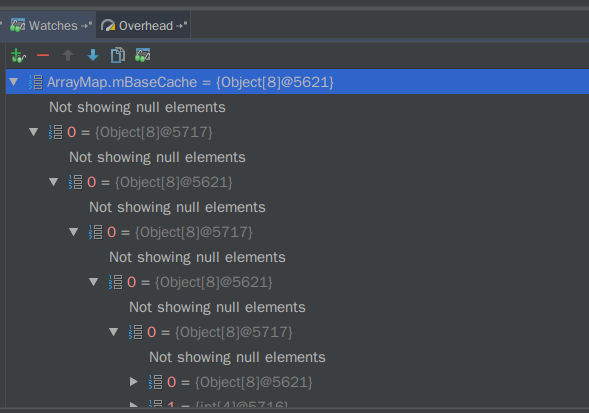``````ArrayMap map4 = new ArrayMap(4);  //取出map2缓存
map4.append("a11", "v11");    //修改map2的array[0
ArrayMap map5 = new ArrayMap(4); // 取出map3缓存
````````````ATAL EXCEPTION: Thread-20
Process: com.gityuan.arraymapdemo, PID: 29003
java.lang.ClassCastException: java.lang.String cannot be cast to java.lang.Object[]
at com.gityuan.arraymapdemo.application.ArrayMap.allocArrays(ArrayMap.java:178)
at com.gityuan.arraymapdemo.application.ArrayMap.<init>(ArrayMap.java:255)
at com.gityuan.arraymapdemo.application.ArrayMap.<init>(ArrayMap.java:238)
at com.gityuan.arraymapdemo.application.MainActivity\$4.run(MainActivity.java:240)
``````

## 四、知识延伸

#### 4.1 HashMap

``````public class HashMap<K,V>
extends AbstractMap<K,V>
implements Map<K,V>, Cloneable, Serializable
{
static final int DEFAULT_INITIAL_CAPACITY = 1 << 4； //默认初始大小为16
static final float DEFAULT_LOAD_FACTOR = 0.75； //默认负载因子
static final int TREEIFY_THRESHOLD = 8;  //当链表个数超过8，则转红黑树

//用于存放数据的核心数组，老版本是HashMapEntry，
transient Node<K,V>[] table；
transient int size; //实际存储的键值对的个数

transient int modCount;  // 用于检测是否存在并发修改，transient修饰则不会进入序列化

}
``````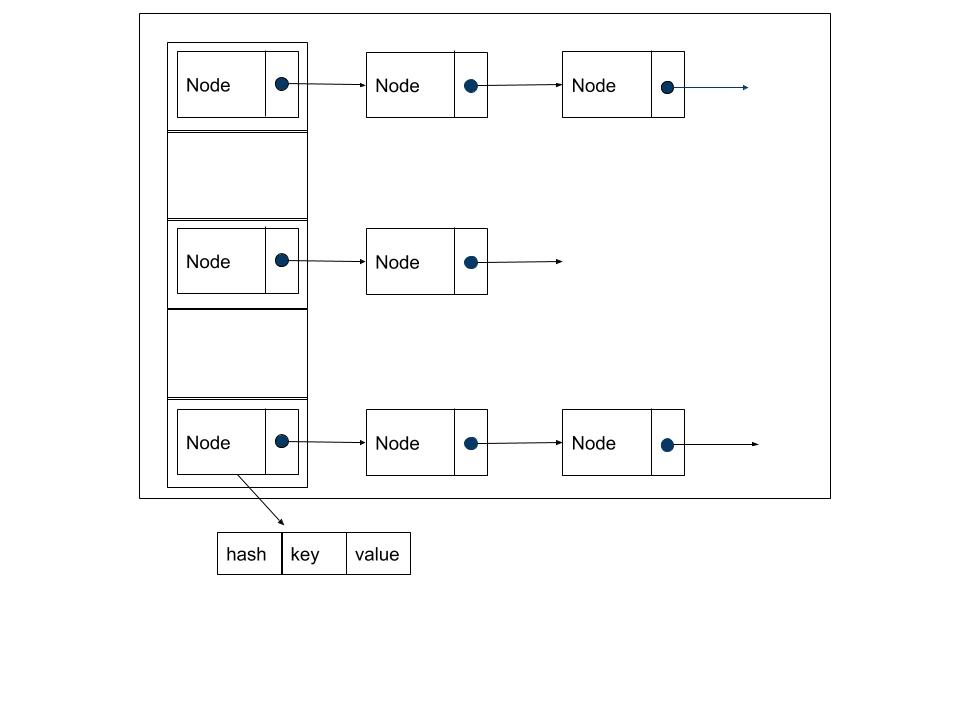HashMap是非线程安全的类，并为了避免开发者错误地使用，在每次增加、删除、清空操作的过程会将modCount次数加1。在一些关键方法内刚进入的时候记录当前的mCount次数，执行完核心逻辑后，再检测mCount是否被其他线程修改，一旦被修改则说明有并发操作，则抛出ConcurrentModificationException异常，这一点的处理比ArrayMap更有全面。

HashMap扩容机制：

• 扩容触发条件是当发生哈希冲突，并且当前实际键值对个数是否大于或等于阈值threshold，默认为0.75*capacity；
• 扩容操作是针对哈希表table来分配内存空间，每次扩容是至少是当前大小的2倍，扩容的大小一定是2^n，； 另外，扩容后还需要将原来的数据都transfer到新的table，这是耗时操作。

#### 4.2 SparseArray

``````public class SparseArray<E> implements Cloneable {
private static final Object DELETED = new Object();
private boolean mGarbage = false; //标记是否存在待回收的键值对

private int[] mKeys;
private Object[] mValues;
private int mSize;
}
``````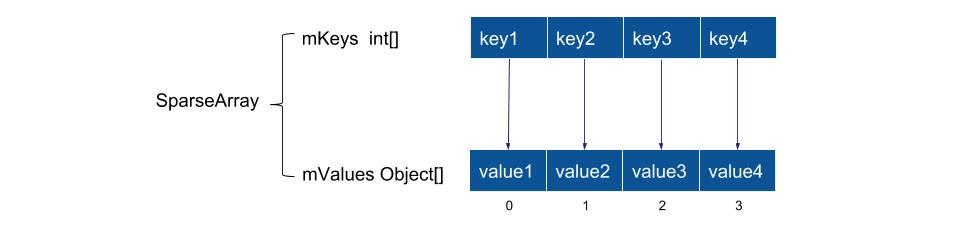SparseArray对应的key只能是int类型，它不会对key进行装箱操作。它使用了两个数组，一个保存key，一个保存value。 从内存使用上来说，SparseArray不需要保存key所对应的哈希值，所以比ArrayMap还能再节省1/3的内存。

SparseArray使用二分查找来找到key对应的插入位置，保证mKeys数组从小到大的排序。

#### 4.2.1 延迟回收

``````public void delete(int key) {
int i = ContainerHelpers.binarySearch(mKeys, mSize, key);
if (i >= 0) {
if (mValues[i] != DELETED) {
mValues[i] = DELETED;  //标记该数据为DELETE
mGarbage = true; // 设置存在GC
}
}
}
``````

``````private void gc() {
int n = mSize;
int o = 0;
int[] keys = mKeys;
Object[] values = mValues;

for (int i = 0; i < n; i++) {
Object val = values[i];
if (val != DELETED) { //将所有没有标记为DELETE的value移动到队列的头部
if (i != o) {
keys[o] = keys[i];
values[o] = val;
values[i] = null;
}
o++;
}
}
mGarbage = false; //垃圾整理完成
mSize = o;
}
``````

#### 4.3 ArraySet

ArraySet也是Android特有的数据结构，用于替代HashSet的，跟ArrayMap出自同一个作者，从源码来看ArraySet跟ArrayMap几乎完全一致，包含缓存机制，扩容机制。唯一的不同在于ArrayMap是一个key-value键值对的集合，而ArraySet是一个集合，mArray[]保存所有的value值，而mHashes[]保存相应value所对应的hash值。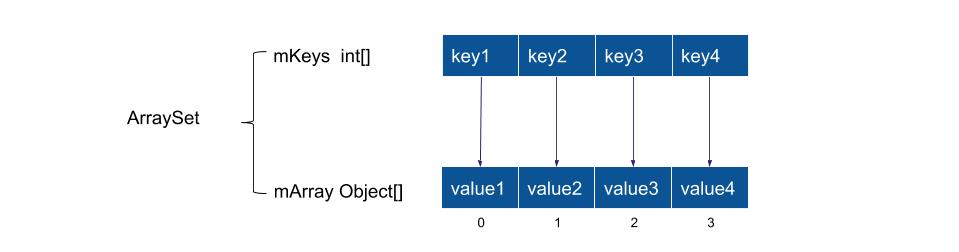``````private void allocArrays(final int size) {
if (size == (BASE_SIZE * 2)) {
...
} else if (size == BASE_SIZE) {
synchronized (ArraySet.class) {
if (sBaseCache != null) {
final Object[] array = sBaseCache;
try {
mArray = array;
sBaseCache = (Object[]) array;
mHashes = (int[]) array;
array = array = null;
sBaseCacheSize--;
return;
} catch (ClassCastException e) {
}
// 从下面这段日志，可以看出谷歌工程师也发现了存在这个问题
// Whoops!  Someone trampled the array (probably due to not protecting
// their access with a lock).  Our cache is corrupt; report and give up.
sBaseCache = null;
sBaseCacheSize = 0;
}
}
}

mHashes = new int[size];
mArray = new Object[size];
}
``````

## 五、总结

• 数据结构
• ArrayMap和SparseArray采用的都是两个数组，Android专门针对内存优化而设计的
• HashMap采用的是数据+链表+红黑树
• 内存优化
• ArrayMap比HashMap更节省内存，综合性能方面在数据量不大的情况下，推荐使用ArrayMap；
• Hash需要创建一个额外对象来保存每一个放入map的entry，且容量的利用率比ArrayMap低，整体更消耗内存
• SparseArray比ArrayMap节省1/3的内存，但SparseArray只能用于key为int类型的Map，所以int类型的Map数据推荐使用SparseArray；
• 性能方面：
• ArrayMap查找时间复杂度O(logN)；ArrayMap增加、删除操作需要移动成员，速度相比较慢，对于个数小于1000的情况下，性能基本没有明显差异
• HashMap查找、修改的时间复杂度为O(1)；
• SparseArray适合频繁删除和插入来回执行的场景，性能比较好
• 缓存机制
• ArrayMap针对容量为4和8的对象进行缓存，可避免频繁创建对象而分配内存与GC操作，这两个缓存池大小的上限为10个，防止缓存池无限增大；
• HashMap没有缓存机制
• SparseArray有延迟回收机制，提供删除效率，同时减少数组成员来回拷贝的次数
• 扩容机制
• ArrayMap是在容量满的时机触发容量扩大至原来的1.5倍，在容量不足1/3时触发内存收缩至原来的0.5倍，更节省的内存扩容机制
• HashMap是在容量的0.75倍时触发容量扩大至原来的2倍，且没有内存收缩机制。HashMap扩容过程有hash重建，相对耗时。所以能大致知道数据量，可指定创建指定容量的对象，能减少性能浪费。
• 并发问题
• ArrayMap是非线程安全的类，大量方法中通过对mSize判断是否发生并发，来决定抛出异常。但没有覆盖到所有并发场景，比如大小没有改变而成员内容改变的情况就没有覆盖
• HashMap是在每次增加、删除、清空操作的过程将modCount加1，在关键方法内进入时记录当前mCount，执行完核心逻辑后，再检测mCount是否被其他线程修改，来决定抛出异常。这一点的处理比ArrayMap更有全面。